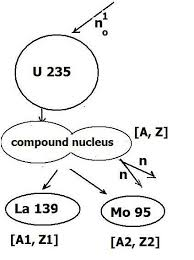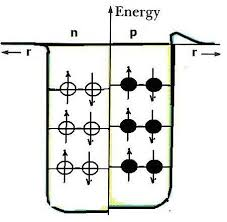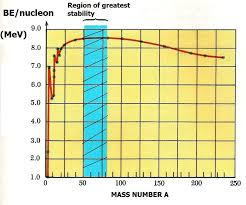## Tuesday, October 14, 2014

### An Introduction to Nuclear Physics (1)

1.The Liquid Drop model

Nuclear models occur under a set of hypotheses, each of which explains some aspect of nuclear behavior. The most basic is perhaps the “liquid drop model” which is used to account for nuclear fission, radioactivity. This is illustrated in Fig, 1 below:Fig. 1: The Liquid Drop model showing U235 Fission

As the name implies, the nucleus is depicted in terms of a “liquid drop”, which analogous to a liquid macroscopic drop, exhibits surface tension and an excitation energy when disturbed.

This model is premised on the exceedingly short range of nuclear forces which requires that nearest neighbor attractions predominate. This is somewhat similar to the type of attraction between the molecules of a liquid which leads to the property of its surface tension.  Thus, in a liquid drop nucleus each nucleon shares its total binding energy with every nucleon.

As the simplified model diagram (for U 235 fission) shows, we expect  a spherical configuration in the minimum energy or unexcited state.  The unstable (compound) nucleus then yields two “daughter nuclei” : La 139 and Mo 95, with two additional neutrons released

The total binding energy initially holding all the nucleons together in the liquid drop nucleus model is comprised of a sum of separate energies, including:  surface or E(s), volume or E(v), and Coulombic repulsion or E(c) – the latter due to the electrostatic repulsion between protons. Thus, the binding energy:

E(B) = E(s) + (E(v) + E(c)

A reduction in any one or all of these constituent energies reduces the overall binding energy and hence the bonding energy between any pair of nucleons. To account for energy release in the fission of heavy nuclei we need only represent the nucleus as a liquid drop and its transition from the unexcited to the excited state as shown in Fig. 1.

Thus, between the spherical and compound nucleus phase, the “drop” may be said to undergo oscillations which lead it to overshoot sphericity in two directions – vertically (converting to an elliptical configuration with semi-major axis in the vertical sense, and horizontally, with semi-major axis in the horizontal sense. It finally reaches a point – the compound nucleus phase – at which the surface tension restoring force is no longer able to contain the long –range Coulombic repulsion force and the inertia of nuclear matter.

At this point, the drop reaches a stage of distortion in which the nucleus splits into two fission fragments, denoted by [A1, Z1] and [A2, Z2]. In order to further assess the nuclear changes, we use what is called a “semi-empirical mass formula” which sets out terms as follows:

(1) fo(Z, A) = 1.008142Z + 1.008982 (A – Z)

Where the Z coefficient is the mass of the hydrogen atom in atomic mass units and the (A – Z) coefficient is the mass of the neutron in amu. The remaining terms listed correct for several effects which contribute to the total nuclear binding energy:

(2) f1 (Z, A) = -a1 A

This term accounts for the binding energy and is essentially proportional to the nuclear mass or the volume of the nucleus.

(3) f2 (Z, A) = +a2 A2/3

This term is a positive correction to the surface area of the nucleus, i.e.  the effect of surface tension energy.

(4) f3 (Z, A) = + a3 (Z2/ A1/3 )

This term accounts for the positive Coulomb energy of the charged nucleus, assumed to be a sphere of radius proportional to  A1/3.

(5) f4 (Z, A) = + a4 (Z  -  A/2)2/ A

This term and the last (6) introduce properties specific to the nucleus. This term is zero for the case of Z = (A – Z) or 2Z = A.

(6) f5 (Z, A) = 0, - f(A), +f(A)

Where  0 is the result when ‘Z even’ pairs with (A – Z) odd, or ‘Z odd’ pairs with (A – Z) even; and –f(A) is the result when Z even pairs with (A – Z) even, and f(A) is the results when Z odd is paired to (A – Z) odd.  The form of f(A) is determined by fitting the data. It is found that the best fit for a simple power law relation is:

f (A) = a5 A - 1/2

Combining all the terms we get:

M(Z, A) = 1.008142Z + 1.008982 (A – Z) -a1 A+ a2 A2/3

+ a3 (Z2/ A1/3 ) + a4 (Z  -  A/2)2/ A +  (-1, 0 , 1) a5 A – 1/2

The parameter values designated are:  a1 = 0.01692,  a2 = 0.01912,  a3 = 0.000763,  a4  = 0.10178, and  a5  = 0.012.

2. The  Nuclear Shell Model:

The shell model of the nucleus treats a different aspect, namely specific energy levels within the nucleus.  To achieve this the nucleons are treated as independent particles, each of which moves in its own spherically symmetrical potential well about 50 MeV deep with rounded edges.Fig. 2: The potential well for the Shell model

The Schrodinger Equation  is then set up for this potential and solved. The solution yields stationary quantum states, somewhat like the states which are associated with electrons in the outer shells of the atom.

Like the electrons occupying atomic shells, the nucleons are fermions, i.e. particles each with spin ½  and therefore obeying the Pauli Exclusion Principle so that no more than two nucleons of the same type can occupy the same energy level (e.g. one with spin +½, the other with spin -½.). This leads to particles pairing up in the nucleus: spin up (+ ½) protons with spin down (-½) protons, and spin up neutrons with spin down neutrons.

The form or expression for the energy of a nucleus is very closely approximated by  the energy associated with a “square well” potential. The Fermi energy in more explicit form (which we will not elaborate upon too much beyond this) is:

EF = 3 2/3 p 4/3 ħ2 r 2/3/ 2M

Where M is the nucleon mass, ħ = h/2p is the adjusted Planck number (h divided by 2p) and r is the nucleon density. Performing the operation with known or estimated values, the result is EF =  30 MeV.   If we assume a spherical nucleus then the radius is just:

R = r o A 1/3

This is also the dimension on either side of the central symmetry line of Fig. 2. The evidence for the shell model includes the following:

i)Particularly stable nuclei are borne out by “closed shells”, based on our observations.

ii) The model predicts (correctly) that the even N (or A – Z) nuclei will be most stable and the odd Z, odd N nuclei the least stable. Experiments bear this out: there are 160 stable nuclides (with even Z, N) and only 4 with odd Z, N.

iii) The model predicts that for even N, Z nuclides the total angular momentum J = 0, and that for odd nuclides it is half-integral. (Borne out by measurements of nuclear magnetic moments.)

Problem: Find the diameter of the oxygen (O 16) nucleus.

Solution:

We apply:  R = r o A 1/3

Where:  r o  =  1.2 x 10 -15 m  is the Bohr radius, and A = 16.  Then:
R = (1.2 x 10 -15 m)  (16)  1/3= 3.0238 x 10 -15 m

And the diameter D = 2R = 2(3.0238 x 10 -15 m)
D = 6.05 x 10 -15 m

The preceding result can also be generalized to a situation in which the ratio of radii (between two nuclei) is sought. In this case, we can write:
R1/ R2  =  [r o A1 1/3] /  [r o A2  1/3]

Or:
R1/ R2 = (A1/ A2) 1/3

Example: How much larger is a copper nucleus than an oxygen nucleus?

Let R1 denote  the radius of the copper nucleus, and R2, oxygen’s. Then: A1 = 64, and A2 = 16. So:
R1/ R2 = (64/16) 1/3 =   4/ 2.51 = 1.587
Thus, the copper nucleus is about 1.587 times the size of the oxygen nucleus.

Problems:

1) Evaluate the terms of the semi-empirical mass formula for the U 238 nucleus,  i.e. if A = 238 and Z = 92. Use this information to find the total mass in atomic mass units (u) and compare it to the standard mass expression. Thence or otherwise, obtain the mass defect and the binding energy as well as the binding energy per nucleon.

2)The sketch graph shown below plots the mass number A vs. the binding energy per nucleon (BE/nucleon) on the vertical axis.Using any models or other explanations, account for the region of greatest stability indicated.

3) A quantum square well potential is  schematically defined according to:It is found that the Schrodinger equation to solve becomes:

2/ 2m ( d
y2/dx2 ) = Ey

Thence:

d
y2/dx2 + 2m/ ħ2 (Ey) = 0

And the quantized energy is found to be:

E n = (h2/ 8m L2) n2
This can be modified to yield a simplified Fermi energy in one dimension by using instead:

E n = (h2  p2/ 8m L2) (N/2)2

Where all the Fermi shell energy levels are presumed occupied up to N/2.  Use this to obtain a simplified estimate of the energy associated with the oxygen nucleus if we assume its shells filled up to N =4 and use an estimate for L as:

R = r o A 1/3

(Take m = 1.7 x 10 -27 kg)

4)(a) Compare the value you obtain in (3) to the actual Fermi (shell) energy for oxygen, if the nuclear density r » 2.3 x 1017 kgm-3.

(b) Write out at least one technique that might be used to find the density of an atomic nucleus. State clearly any assumptions made and how you quantify them.

5)(a) Find the ratio of the helium nucleus radius to that of the uranium 238 nucleus.

(b) Estimate, using any technique you can think of, the ratio of the nuclear densities for part (a).

6) An element has mass number A = 202 and atomic number Z = 80.

a) Find the diameter of the nucleus and how many times it is greater than that of hydrogen.
b) Find the mass defect D M for this nucleus

c) From the result in (b) obtain the binding energy and the binding energy per nucleon, EB/ A.  Using the graph on the opposite page, mark the location of EB/ A.